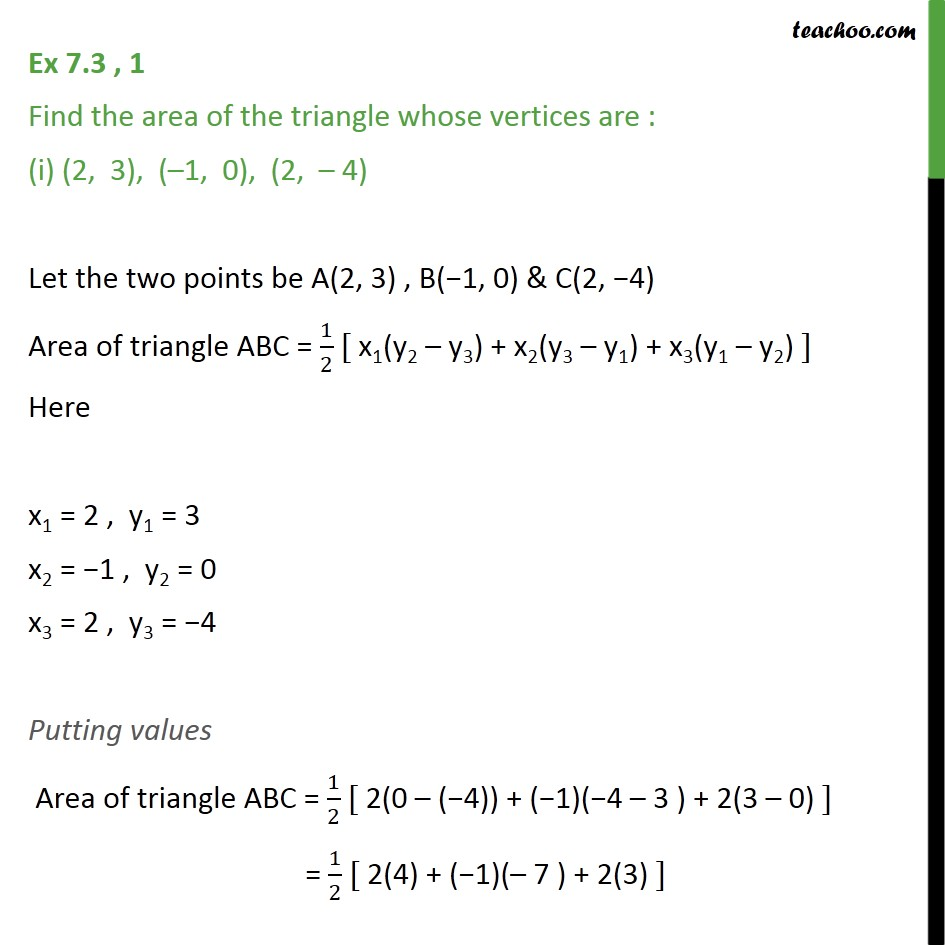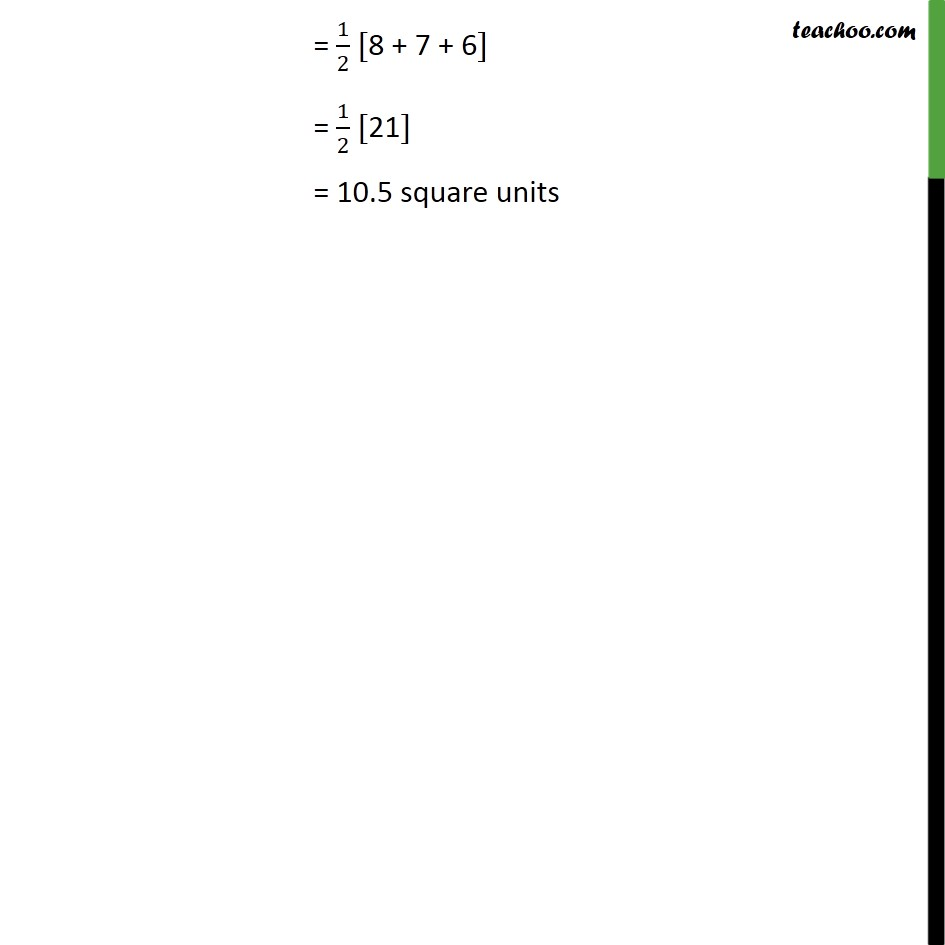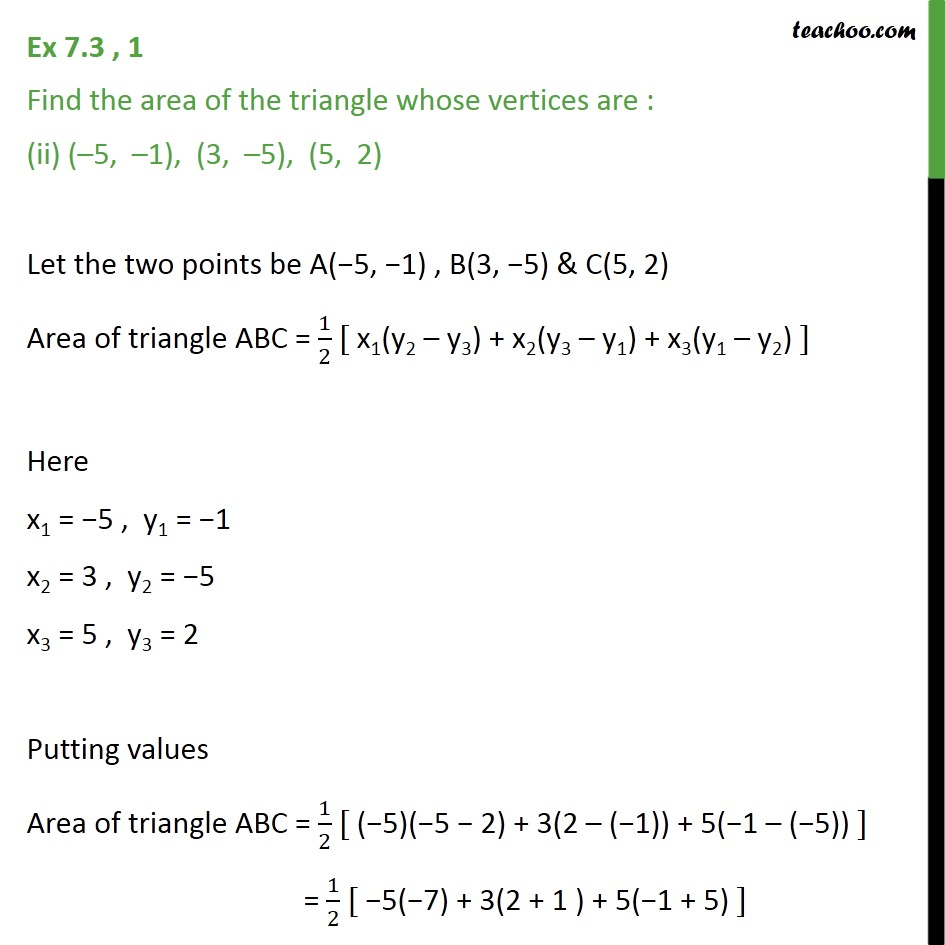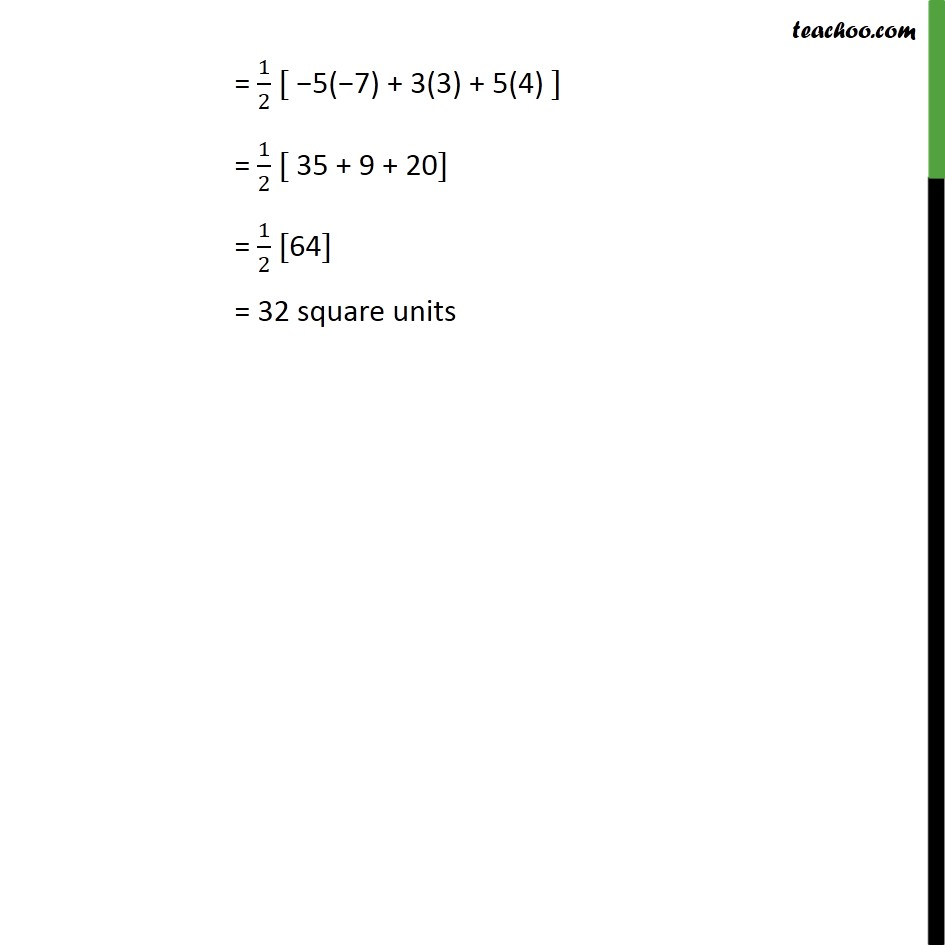Learn all Concepts of Chapter 7 Class 10 (with VIDEOS). Check - Coordinate Geometry - Class 101. Chapter 7 Class 10 Coordinate Geometry
2. Serial order wise
3. Ex 7.3

Transcript

Ex 7.3 , 1 Find the area of the triangle whose vertices are : (i) (2, 3), ( 1, 0), (2, 4) Let the two points be A(2, 3) , B( 1, 0) & C(2, 4) Area of triangle ABC = 1/2 [ x1(y2 y3) + x2(y3 y1) + x3(y1 y2) ] Here x1 = 2 , y1 = 3 x2 = 1 , y2 = 0 x3 = 2 , y3 = 4 Putting values Area of triangle ABC = 1/2 [ 2(0 ( 4)) + ( 1)( 4 3 ) + 2(3 0) ] = 1/2 [ 2(4) + ( 1)( 7 ) + 2(3) ] = 1/2 [8 + 7 + 6] = 1/2  = 10.5 square units Ex 7.3 , 1 Find the area of the triangle whose vertices are : (ii) ( 5, 1), (3, 5), (5, 2) Let the two points be A( 5, 1) , B(3, 5) & C(5, 2) Area of triangle ABC = 1/2 [ x1(y2 y3) + x2(y3 y1) + x3(y1 y2) ] Here x1 = 5 , y1 = 1 x2 = 3 , y2 = 5 x3 = 5 , y3 = 2 Putting values Area of triangle ABC = 1/2 [ ( 5)( 5 2) + 3(2 ( 1)) + 5( 1 ( 5)) ] = 1/2 [ 5( 7) + 3(2 + 1 ) + 5( 1 + 5) ] = 1/2 [ 5( 7) + 3(3) + 5(4) ] = 1/2 [ 35 + 9 + 20] = 1/2  = 32 square units

Ex 7.3